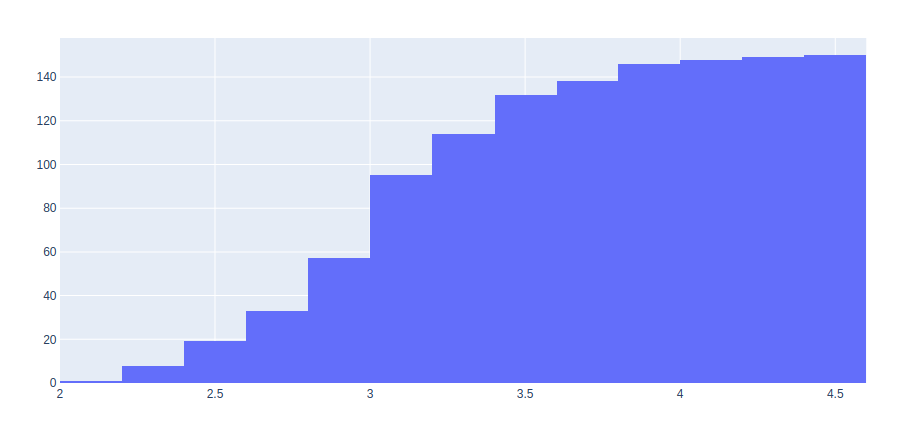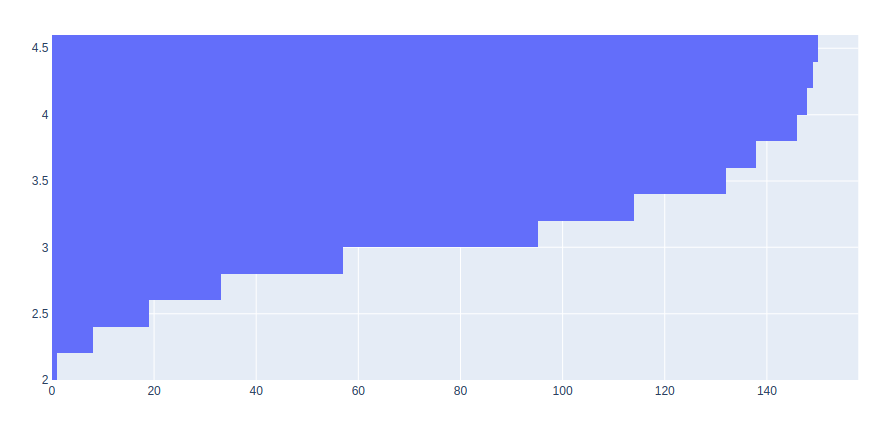GeeksforGeeks App
Open AppBrowser
Continue

# How to create a Cumulative Histogram in Plotly?

Plotly is a Python library that is used to design graphs, especially interactive graphs. It can plot various graphs and charts like histogram, barplot, boxplot, spreadplot, and many more. It is mainly used in data analysis as well as financial analysis. plotly is an interactive visualization library.

## Creating cumulative histogram

A cumulative histogram is a histogram that counts the cumulative cases over the range of cases. It can be created by passing True to the cumulative_enabled parameter.

Example 1: Vertical cumulative histogram

## Python3

 `import` `plotly.express as px ``import` `plotly.graph_objects as go ``   ` `df ``=` `px.data.iris() ``   ` `fig ``=` `go.Figure(data``=``[go.Histogram(x``=``df[``'sepal_width'``], ``                                   ``cumulative_enabled``=``True``)]) ``fig.show()`

Output:Example 2: Horizontal cumulative histogram

## Python3

 `import` `plotly.express as px ``import` `plotly.graph_objects as go ``   ` `df ``=` `px.data.iris() ``   ` `fig ``=` `go.Figure(data``=``[go.Histogram(y``=``df[``'sepal_width'``], ``                                   ``cumulative_enabled``=``True``)]) ``fig.show()`

Output:My Personal Notes arrow_drop_up